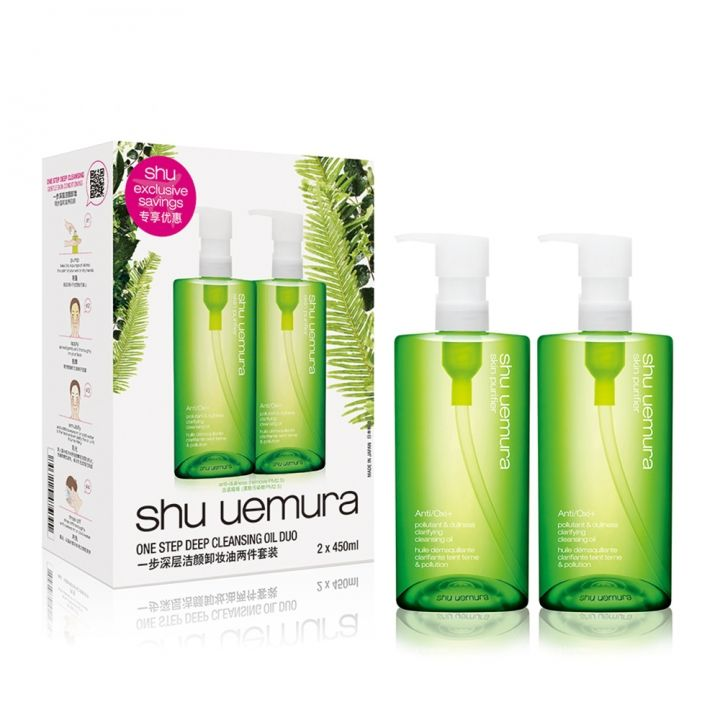388 搜索結果 / ITEMS

# 保養特惠組

#### 搜索過濾

• 折扣
• 保養
• 彩妝
• 香氛
• 女仕
• 男仕
• 數位
• 旅行居家
• 伴手禮

• Hello Kitty粉紅旋風
• 女仕折扣專區5折起
• 男仕折扣專區5折起
• 台灣鳯梨酥買多送多
• 樂高積木任選2件9折
• 全館飾品獨享品牌禮
• 100%台灣嚴選茶禮盒
• 臉部保養
• 身體護理
• 手足護理
• 頭髮護理
• 男仕保養
• 保養特惠組
• 免稅獨賣
• 寶寶護理
• 深刻台灣
• 臉部彩妝
• 眼部彩妝
• 唇部彩妝
• 彩妝特惠組
• 彩妝工具及其他
• 免稅獨賣
• 手足身體彩妝
• 女性香水
• 男性香水
• 中性香水
• 香水特惠組
• 居家香氛
• 免稅獨賣
• 免稅獨賣
• 女用包
• 配件
• 飾品
• 珠寶
• 女錶
• 女裝
• 女鞋
• 旅行用品
• 免稅獨賣
• 男用包
• 配件
• 飾品
• 男錶
• 男裝
• 男鞋
• 旅行用品
• 手機與配件
• 電腦與平板
• 相機與攝影
• 遊戲與主機
• 網卡與電源
• 智慧穿戴
• 音響耳機
• 旅行頸枕
• 旅行用品
• 美容家電
• 生活家電
• 生活用品
• 幼兒玩具/用品
• 巧克力
• 糖果/餅乾/零嘴
• 糕酥禮盒
• 咖啡/茶
• 台灣伴手禮
• 世界伴手禮
• 台灣文創與藝術
• 玩具模型
• 免稅獨賣

• 臉部清潔
• 導入液
• 化妝水
• 精華液
• 乳液/乳霜/凝膠
• 眼唇護理
• 面膜
• 防曬
• 護理油
• 臉部特殊護理
• 美容用品
• 身體清潔
• 身體乳液/乳霜
• 體香劑
• 美胸
• 身體防曬
• 美體產品
• 身體特殊護理
• 護手霜
• 護足霜
• 頭髮清潔
• 頭髮潤絲
• 頭髮特殊護理
• 美髮造型
• 清潔/刮鬍
• 男仕化妝水
• 男仕精華液
• 男仕乳液/乳霜
• 男仕眼唇護理
• 男仕特殊護理
• 寶寶清潔
• 寶寶乳液/乳霜
• 其他寶寶護理
• 隔離霜/飾底乳
• 粉底
• 粉餅
• 遮瑕
• 蜜粉
• 腮紅
• BB霜/CC霜
• 眉筆/眉粉/染眉膏
• 眼線
• 眼影
• 睫毛膏
• 唇膏
• 唇筆
• 唇釉/唇蜜/唇露
• 指甲油/美甲
• 套組
• 女性Parfum 香精
• 女性E.D.P. 香水
• 女性E.D.T. 淡香水
• 女性Cologne 古龍水
• 女性香水綜合套組
• 其他女性香水
• 男性Parfum 香精
• 男性E.D.P. 香水
• 男性E.D.T. 淡香水
• 男性Cologn 古龍水
• 男性香水綜合套組
• 中性E.D.T. 淡香水
• 中性香水綜合套組
• 其他中性香水
• 迷你香水組
• 綜合套組
• 後背包
• 肩背包
• 斜背包
• 手提包
• 手拿包
• 腰包
• 小皮件
• 太陽眼鏡
• 帽子/圍巾
• 皮帶
• 其它配件
• 耳環
• 戒指
• 手鍊手環
• 項鍊與項墜
• 髮飾
• 其它飾品
• 耳環
• 項鍊與項墜
• 手鍊手環
• 戒指
• 金飾
• 精品錶
• 休閒錶
• 兒童錶
• 對錶
• 手錶配件
• 旅行袋
• 旅行箱
• 兒童箱包
• 其它旅行用品
• 後背包
• 側肩包
• 斜背包
• 手提包
• 手拿包
• 腰包
• 小皮件
• 太陽眼鏡
• 帽子/圍巾
• 皮帶
• 其它配件
• 領帶/袖扣
• 耳環
• 戒指
• 手鍊手環
• 項鍊與項墜
• 精品錶
• 休閒錶
• 兒童錶
• 對錶
• 手錶配件
• 旅行袋
• 旅行箱
• 兒童箱包
• 其它旅行用品
• Apple專區
• ASUS專區
• Samsung專區
• Apple專區
• 筆記型電腦
• 平板電腦
• 單眼/DSLR
• 一般相機
• 攝影機與空拍機
• 旅行袋/包/箱
• 兒童背包/箱
• 證件夾
• 網卡與電源
• 其它旅行用品
• 吹風機與美髮造型
• 洗臉機與美膚儀
• 其他美容家電
• 吸塵器
• 刮鬍刀
• 電動牙刷
• 其他生活家電
• 食物罐與保溫瓶
• 其他生活用品
• 木製家飾
• 琉璃家飾
• 音樂水晶球
• 飾品
• 護膚清潔
• 其他文創商品
• 漫威與DC
• 星際大戰
• 樂高玩具
• 其它玩具模型
• 冰雪奇緣

• 預設
• 時間 (近到遠)
• 價格 (高到低)
• 價格 (低到高)
• 品牌名稱 (A-Z)
• 品牌名稱 (Z-A)

• 顯示 12 樣
• 顯示 48 樣
• 顯示全部

#### 數量顯示

NT\$ 4,000

NT\$ 5,090

NT\$ 15,300

NT\$ 6,900

NT\$ 1,950

NT\$ 4,130

NT\$ 10,300Shu Uemura 植村秀 2017 DUO Anti/Oxi+ pollutant & dullness clarifying cleansing oil 植物精萃潔顏油兩件特惠組

NT\$ 4,350

NT\$ 2,210

NT\$ 2,550